Balbharti Maharashtra Board Class 5 Maths Solutions Chapter 11 Problems on Measurement Problem Set 47 Textbook Exercise Important Questions and Answers.

## Maharashtra State Board Class 5 Maths Solutions Chapter 11 Problems on Measurement Problem Set 47

Question 1.
For his birthday, Ajay gave 20 l 450 ml of milk to the children in an Ashramshala and 28 l 800 ml to the children in an orphanage. How much milk did Ajay donate?
Solution:

 l ml 1 2 0 + 2 8 4 5 0 8 0 0 4 9 2 5 0

450 ml + 800 ml
= 1250 ml
= 11 + 250 ml

∴ Ajay donated 49 l 250 ml milkQuestion 2.
Under the Rural Cleanliness Mission, college students cleaned 1 km 750m of a village road that is 2 km 575m long. How much remained to be cleaned?
Solution:

 km m 1 1 5 7 5 2 – 1 5 7 5 7 5 0 0 8 2 5

750 m cannot be subtracted from 575 m. So, convert 1 km = 1000 m.
∴ 825 m remained to be cleaned

Question 3.
Babhulgaon used 21,250 liters of treated waste water in the fields. Samvatsar used 31,350 litres of similar water. How much treated waste water was used in all?
Solution:
2 1 2 5 0 litres Babhulgaon used
+ 3 1 3 5 0 litres Samvatsar used
___________
5 2 6 0 0
___________

∴ 52,600 litres of waste water used in allQuestion 4.
If half a litre of milk costs 22 rupees, how much will 7 litres cost?
Solution:
$$\begin{array}{l}\frac{1}{2}+\frac{1}{2}=\frac{1+1}{2}=\frac{2}{2}=1 \text { litre } \\ 22+22=₹ 44\end{array}$$
That is, 1 litre cost ₹ 44
∴ 7 litres costs 44 x 7 = ₹ 308
∴ 7 litres costs ₹ 308

Question 5.
If the speed of a motorcycle is 40 km per hour, how far will it travel in an hour and a quarter?
Solution:
Hour and quarter = 1 + $$\frac{1}{4}$$ hours
= 40 km + $$\frac{1}{4}$$ x 40 km
= 40 km + 10 km
= 50 km
∴ Motorcycle will travel in a hour and a quarter 50 km

Question 6.
If a man walks at a speed of 4 kmph, how long will it take him to walk 3 km?
Solution:
1 km = 1000 m
4 km in 1 hour, 4 km in 60 minutes
That is
2 km in 30 minutes
+ 1 km in 15 minutes
_______________________
3 km in 45 minutes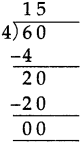That is 1 km in 15 minutes Hence, 3
km in 15 x 3 = 45 min

∴ 3 km in 15 x 3 = 45 minQuestion 7.
If a rickshaw travels at a speed of 30 kmph, how far will it travel in three quarters of an hour?
Solution:
30 kmph means
In 60 minutes 30 km and 30 minutes 15 km
and 15 minutes $$\frac{15}{2}=\frac{15 \times 5}{2 \times 5}=\frac{75}{10}$$ = 7.5 km
∴ In 45 minutes 15 km + 7.5 km = 22.5 km

Question 8.
During Cleanliness Week, children cleaned the public park in their town. They collected three quarter kilograms of plastic bags and five and a half kilograms of other garbage. How much garbage did they collect in all?
Solution: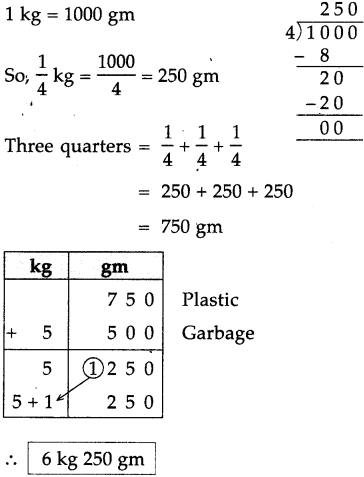Question 9.
If one shirt needs 2 m 50cm of cloth, how much cloth do we need for 5 shirts?
Solution: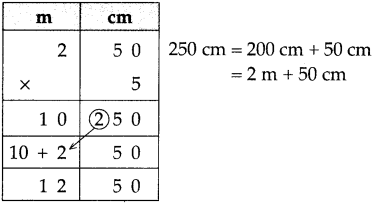∴ 12 m 50 cm cloth needs

Question 10.
If a car travels 60 km in an hour, how far will it travel in
(1) 2 hours?
(2) 15 minutes?
(3) half an hour?
(4) three and a half hours?
Solution:
60 kmph
In 60 minutes 60 km
Hence, 1 minute 1 km
(1) 2 hours = 2 x 60 = 120 km
(2) In 15 minutes = 15 km
(3) In half an hour 60 ÷ 2 = 30 km
(4) In three and half hours
= 3 x 60 + 30
= 180 + 30
= 210 km

∴ (1) 120 km
(2) 15 km
(3) 30 km
(4) 210 kmQuestion 11.
If one gold bangle is made from 12 grams 250 milligrams of gold, how much gold will be needed to make 8 such bangles? (1000mg = 1 g)
Solution: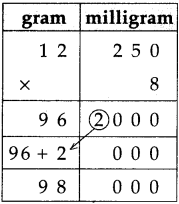∴ 98 grams gold needed

Question 12.
How many pouches of 20g cloves each can be made from 1 kg 240g of cloves?
Solution:
1 kg 240 gm
= 1000 gm + 240 gm
= 1240 gm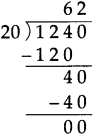Question 13.
Seema’s mother bought 2m 70cm of cloth for a kurta and 2 m 40cm for a shirt. How much cloth did she buy in all?
Solution:
70 cm + 40 cm
= 110 cm
= 1 m 10 cm

 m cm 1 2 + 2 7 0 4 0 5 1 0

cloth for Kurta
cloth for Shirt

∴ 5 m 10 cm cloth in allQuestion 14.
A water tank holds 125 l of water. If 97 l 500 ml of the water is used, how much water remains in the tank?
Solution:
1 litre = 1000 ml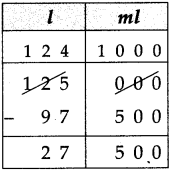water tank holds
water used
water remain

∴ 27 l 500 ml water remain in tank

Question 15.
Harminder bought 57 kg 500g of wheat from one shop and 36 kg 800 g of wheat from another shop. How much wheat did he buy altogether?
Solution: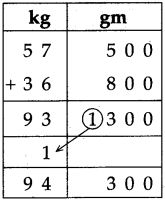bought from 1 shop
bought from another shop
500 + 800 = 1300 gm
= 1000 + 300
= 1 kg 300 gm

∴ 94 kg 300 gm bought altogetherQuestion 16.
Renu took part in a 100m race. She tripped and fell after running 80 m 50 cm. How much distance did she have left to run?
Solution:

 m cm 9 9 1 0 0 1 0 0 – 8 0 0 0 5 0 1 9 5 0

Borrow l m = 100 cm
So, 100 m = 99 m + 100 cm
Total distance to run
Distance covered
Distance left to run

∴ 19 m 50 cm distance left to run

Question 17.
A sack had 40kg 300 grams of vegetables. There were 17kg 700 g potatoes, 13 kg 400g cabbage and the rest were onions. What was the weight of the onions?
Solution: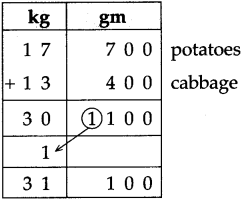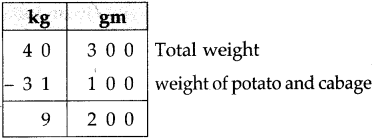∴ Weight of onions is 9 kg 200 gmQuestion 18.
One day, Gurminder Singh walked 3 km 750m and Parminder Singh walked 2km 825m. Who walked farther and by how much?
Solution: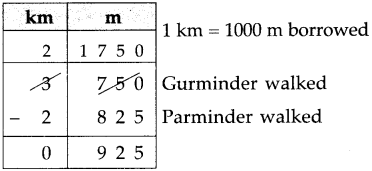∴ Gurminder walked more by 925 metres

Question 19.
Suresh bought 3kg 250g of tomatoes, 2 kg 500g of peas and 1kg 750g of cauliflower. How much was the total weight of the vegetables he bought?
Solution: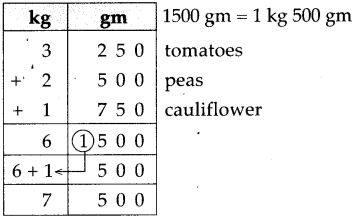∴ Total weight 7 kg 500 gm

Question 20.
Jalgaon, Bhusawal, Akola, Amravati and Nagpur lie serially on a certain route. The distances between Akola and these other places are given below.

Use them to make word problems and solve the problems.
Amravati – 95 km, Bhusawal – 154 km,
Nagpur – 249 km, Jalgaon – 181 km
Solution:
(1) What is the distance between Bhusaval and Nagpur?
249 km – 154 km = 95 km

∴ The distance between Bhusaval and Nagpur is 95 km(2) What is the distance between Amravati and Jalgaon?
181 km – 95 km = 86 km

∴ The distance between Amravati and Jalgaon is 86 km.

Question 21.
Complete the following table and prepare the total bill.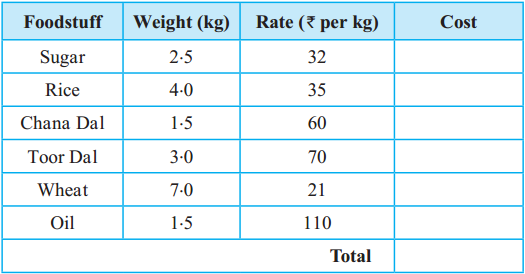Solution: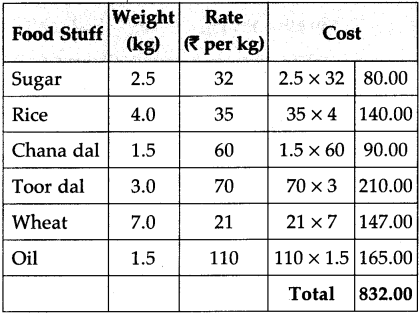Activity

• You have 1 kg of potatoes. Find out which other ingredients you will need to make potato vadas and approximately how much of each ingredient you will need. Also find out approximately how much each ingredient will cost and how many vadas you will be able to make.
• Fix a 1 m long stick in an open field. Measure the shadow of the stick at 9:00 in the morning, at 12:00 noon, at 3:00 in the afternoon and at 5:00 in the evening. Observe at which time of the day the shadow is shortest and at what time, it is longest.
• Measure the length of a pen refill.

Question 1.
One can contains 30 l 560 ml of milk, while second contains 251890 ml of milk and third one contains 20 l 760 ml of milk. How much milk is there in the three cans together?
Solution: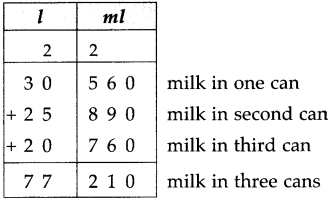∴ 77 l 210 ml total milkQuestion 2.
(1) ₹ 13, 85 paise + ₹ 16, 40 paise
(2) 15 kg 280 gm + 18 kg 920 gm
(3) 24 l 690 ml + 25 l 780 ml
(4) 22 km 750 m + 27 km 500 m
(5) 17 m 40 cm + 19 m 85 cm
(6) 38 cm 8 mm + 17 cm 2 mm
(7) 10 km 950 m + 15 km 125 m
(8) 83 kg 468 gm + 109 kg 532 gm
(1) ₹ 30, 25 paise
(2) 34 kg 200 gm
(3) 50 1 470 ml
(4) 50 km 250 m
(5) 37 m 25 cm
(6) 56 cm
(7) 26 km 75 m
(8) 193 kgQuestion 3.
Subtract the following:
(1) ₹ 21, 30 paise – ₹ 13, 80 paise
(2) 16 kg 130 gm – 9 kg 250 gm
(3) 9 l 350 ml – 5 l 470 ml’
(4) 41 m 10 cm – 14 m 40 cm
(5) 38 km 175 m – 20 km 365 m
(6) 27 cm 5 mm – 11 cm 8 mm
(7) 28 km 725 m – 13 km 590 m
(8) 380 kg – 232 kg 730 gm
(1) ₹ 7, 50 paise
(2) 6 kg 880 gm
(3) 51 880 ml
(4) 26 m 30 cm
(5) 17 km 810 m
(6) 15 cm 7 mm
(7) 15 km 135 m
(8) 147 kg 270 gm

Question 4.
Fill in the blanks:
(1) 1250 m = …………………… km …………………… m
(2) 2.5 m = …………………… m …………………… cm
(3) 3 l 50 ml = …………………… ml
(4) ₹ 2.5 = …………………… paise
(1) 1 km 250 m
(2) 2 m 50 cm
(3) 3050 ml
(4) 250 paiseQuestion 5.
(A) Match the following:

 ‘A’ ‘B’ (1) Potato 3.5 kg, rate per kg ₹ 12 (a) ₹ 40 (2) Onion 2 kg, rate per kg ₹ 20.50 (b) ₹ 42 (3) Vegetables 2.5 kg, rate per kg ₹ 16 (c) ₹ 39 (4) Others 6.5 kg, rate per kg ₹ 6 (d) ₹ 41

(1 – b),
(2 – d),
(3 – a),
(4 – c)(B) Match the following:

 ‘A’ ‘B’ (1) Half metre (a) 5 cm (2) Half kilometre (b) 50 cm (3) 50 millimetre (c) 500 cm (4) 5 kilometre (d) 500 m (5) 5 metre (e) 5000 m